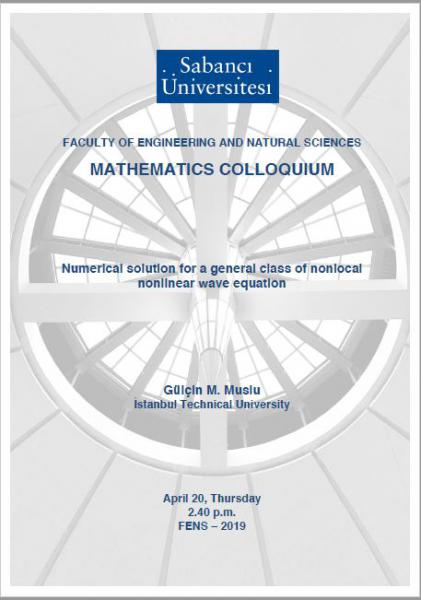## Mathematics Colloquium: Numerical solution for a general class of non...

### Mathematics Colloquium: Numerical solution for a general class of non...Title: Numerical solution for a general class of nonlocal nonlinear wave equation

Speaker: Gülçin M. Muslu

Date/Time: Thursday, 20 April 201714.40 pm.

Location: FENS 2019

Abstract: In this talk, we consider a general class of  nonlocal  nonlinear wave equation arising in one-dimensional nonlocal elasticity. The model involves a convolution operator with a general kernel function whose Fourier transform is nonnegative. We propose a Fourier collocation numerical method for the nonlocal nonlinear wave equation. We first test our scheme for some  examples of nonlocal nonlinear wave equation, such as generalized improved Boussinesq  equation and the higher-order Boussinesq equation which arise from the  suitable choices of the kernel function.  We prove the convergence of the semi-discrete schemes in an appropriate energy spaces. We consider  three test problems concerning the propagation of a single solitary wave,  the interaction of two solitary waves and a solution that blows-up in finite time. We then extend our convergence proofs to the  nonlocal nonlinear wave equation including more general kernels. To understand the structural properties of the solutions of nonlocal nonlinear wave equation, we present some numerical results illustrating the effects of  the kernel function on the solutions.
This is a joint work with H. Borluk and G. Topkarci.
This work has been supported by the Scientific and Technological Research Council of Turkey (TUBITAK) under the project MFAG-113F114.
Contact: Yasemin Şengül